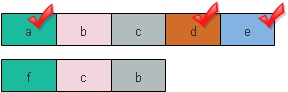# array_diff(): Difference between two arrays

This function takes two or more arrays as input and then returns the result array as difference.
``\$new_array = array_diff (\$array1, \$array2,....);``
Returns all elements of \$array1 which are not present in \$array2 (\$new_array=\$array1 without \$array2).

## Example with two arrays``````\$first=array('a','b','c','d','e');
\$second=array('f','c','b');
\$result=array_diff(\$first,\$second);
while (list (\$key, \$val) = each (\$result)) {
echo "\$key -> \$val <br>";
}``````
Output is here.
``````0 -> a
3 -> d
4 -> e ``````
Keys of the first index are retained. ( no re-indexing done here)

## Without any common element

``````\$first=array('a','b','c','d','e');
\$second=array('f','g','h');
\$result=array_diff(\$first,\$second);
while (list (\$key, \$val) = each (\$result)) {
echo "\$key -> \$val ";
}``````
Output is here
``````0 -> a
1 -> b
2 -> c
3 -> d
4 -> e ``````
Keys of the first array is retained without any re-indexing.

## associative array with literal keys

``````\$first=array('Fruits1' =>'Banana','Fruits2'=>'Mango','Fruits3'=>'Apple');
\$second=array('new_fruit1'=> 'Strawberry','new_fruit2'=>'Mango');
\$result=array_diff(\$first,\$second);
while (list (\$key, \$val) = each (\$result)) {
echo "\$key -> \$val <br>";
}``````
Output is here
``````Fruits1 -> Banana
Fruits3 -> Apple ``````
For example let us use one array a1 and store text entered by user in a web form after converting the text string to an array. Now to prevent spammers posting URL or posting of some bad words we will develop another array a2 where all negative words we will store. These words we don't want to be present in the first ( a1) array.

Here is the example of how the array_diff works

``````\$a1=array("first", "second", "third","fourth","fifth");
\$a2=array("second","fourth","sixth");

\$new_array=array_diff(\$a1,\$a2);

while (list (\$key, \$val) = each (\$new_array)) {
echo "\$key -> \$val <br>";
}``````
The output of above code is here

0 -> first
2 -> third
4 -> fifth

## Array_diff() and array_diff_key()

Using array_diff() comparison is done using the values, in case of array_diff_key() comparison is done using the keys only.Subscribe to our YouTube Channel here

## Subscribe

* indicates required
Subscribe to plus2netplus2net.com

 MAZHAR HUSSAIN 08-08-2010 PLEASE SEND ME SIR!DIFFERENCE BETWEEN FUNCTION AND ARRAY?

Post your comments , suggestion , error , requirements etc here .